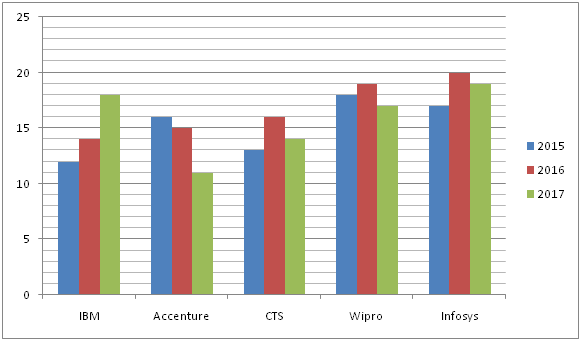# SBI Clerk Pre Quantitative Aptitude (Day-45)

Dear Aspirants, Our IBPS Guide team is providing new series of Quantitative Aptitude Questions for SBI Clerk Prelims 2020 so the aspirants can practice it on a daily basis. These questions are framed by our skilled experts after understanding your needs thoroughly. Aspirants can practice these new series questions daily to familiarize with the exact exam pattern and make your preparation effective.

Start Quiz

Ensure Your Ability Before the Exam – Take SBI Clerk 2020 Prelims Free Mock Test

Data Interpretation

Directions (1 – 5): Study the following information carefully and answer the given questions?

The following bar graph shows the total number of employees (In thousands) working in 5 different MNC companies in 3 different years.1) Find the difference between the total number of employees working in IBM and CTS together in the year 2015 to that of total number of employees working in Accenture and Wipro in the year 2017?

a) 3000

b) 4000

c) 2000

d) 5000

e) None of these

2) Find the ratio between the total number of employees working in Wipro and Infosys together in the year 2016 to that of total number of employees working in IBM and Accenture together in the same year?

a) 15: 7

b) 23: 14

c) 39: 29

d) 57: 43

e) None of these

3) If the ratio between the total number of male to that of female employees working in Accenture in the year 2015 and that in CTS in the year 2017 is 3 : 5 and 4 : 3 respectively, then find the sum of the total number of female employees working in Accenture in the year 2015 and the total number of male employees working in CTS in the year 2017?

a) 22000

b) 20000

c) 24000

d) 18000

e) None of these

4) Find the difference between the average number of employees working in Accenture to that of Wipro in all the given years together?

a) 5000

b) 4000

c) 3000

d) 6000

e) None of these

5) Total number of employees working in Accenture, CTS and Infosys together in the year 2016 is approximately what percentage of total number of employees working in IBM in all the given years together?

a) 130 %

b) 142 %

c) 116 %

d) 95 %

e) 158 %

Simplification

Directions (6 – 10): What value should come in the place of (?) in the following questions?

6) 80 % of 240 = ? + 119 – 3 * √81

a) 90

b) 100

c) 80

d) 70

e) 60

7) 3(5/8) = 3(1/8) * 3(y/8)

a) 3

b) 5

c) 8

d) 4

e) 6

8) √841 + ? * 5 = 8 (3/5) * 65

a) 105

b) 106

c) 107

d) 104

e) 108

9)  ? ÷ 30 + 12 % of 125 = 4(1/2) * 7

a) 490

b) 495

c) 500

d) 505

e) 510

10) (780 ÷ 4) – 12 * 6 + √729 = ?

a) 150

b) 120

c) 180

d) 210

e) 90

Directions (1-5) :

The total number of employees working in IBM and CTS together in the year 2015

= > 12000 + 13000 = 25000

The total number of employees working in Accenture and Wipro in the year 2017

= > 11000 + 17000 = 28000

Required difference = 28000 – 25000 = 3000

The total number of employees working in Wipro and Infosys together in the year 2016

= >19000 + 20000 = 39000

The total number of employees working in IBM and Accenture together in the year 2016

= >14000 + 15000 = 29000

Required ratio = 39000: 29000 = 39: 29

The total number of female employees working in Accenture in the year 2015

= > 16000 * (5/8) = 10000

The total number of male employees working in CTS in the year 2017

= > 14000 * (4/7) = 8000

Required sum = 10000 + 8000 = 18000

The average number of employees working in Accenture in all the given years together

= > (16000 + 15000 + 11000)/3

= > 42000/3 = 14000

The average number of employees working in Wipro in all the given years together

= > (18000 + 19000 + 17000)/3

= > 54000/3 = 18000

Required difference = 18000 – 14000 = 4000

Total number of employees working in Accenture, CTS and Infosys together in the year 2016

= > (15000 + 16000 + 20000) = 51000

Total number of employees working in IBM in all the given years together

= > (12000 + 14000 + 18000) = 44000

Required % = (51000/44000) * 100 = 115.909 % = 116 %

Directions (6-10) :

80 % of 240 = ? + 119 – 3 * √81

192 = ? + 119 – 27

? = 100

3(5/8) = 3(1/8) * 3(y/8)

35/8 – 1/8 = 3(y/8)

4/8 = y/8

y = 4

√841 + ? * 5 = 8 (3/5) * 65

29 + ? * 5 = 43/5 * 65

? * 5 = 530

? = 106

? ÷ 30 + 12 % of 125 = 4 (1/2) * 7

?/30 + 15 = 31.5

?/30 = 16.5

? = 495

(780 ÷ 4) – 12 * 6 + √729 = ?

195 – 72 + 27 = ?

150 = ?

 Check Here to View SBI Clerk Prelims 2020 Quantitative Aptitude Questions Day 44 Day 43 Day 42 Click Here for SBI Clerk 2020 – Detailed Exam Notification